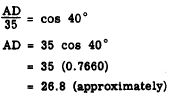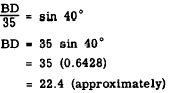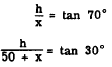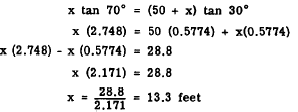Custom SearchOBLIQUE TRIANGLES Oblique triangles were defined in chapter 17 of this training course as triangles which contain no right angles. A natural approach to the solution of problems involving oblique triangles is to construct perpendicular lines and form right triangles which subdivide the original triangle. Then the problem is solved by the usual methods for right triangles. DIVISION. INTO RIGHT .TRIANGLES The oblique triangle ABC in figure 19-18 has been divided into two right triangles by drawing line BD perpendicular to AC. The length of AC is found as follows: 1. Find the length of AD.Figure 19-18.-Finding the unknown parts of an oblique triangle. CAUTION: A careless appraisal of this problem may lead the unwary trainee to represent the ratio AC/AB as the cosine of 40. This error is avoided only by the realization that the trigonometric ratios are based on RIGHT triangles. 2. In order to find the length of DC, first calculate BD.3. Find the length of DC4. Add AD and DC to find AC.SOLUTION BY SIMULTANEOUS EQUATIONS A typical problem in trigonometry is the determination of the height of a point such as B in figure 19-19.Figure 19-19.-Calculation of unknown quantities by means of oblique triangles. Suppose that point B is the top of a hill, and point D is inaccessible. Then the only measurements possible on the ground are those shown in figure 19-19. If we let h represent BD and x represent CD, we can set up the following system of simultaneous equations:Solving these two equations for h in terms of x, we have         h= x tan 70 and         h = (50 + x) tan 30 Since the two quantities which are both equal to h must be equal to each other, we haveKnowing the value of x, it is now possible to compute h as follows:Practice problems: 1. Find the length of side BC in figure 19-20 (A). 2. Find the height of point B above line AD in figure 19-20 (B). Answers: 1. 21.3 feet  2. 41.7 feetIntegrated Publishing, Inc. - A (SDVOSB) Service Disabled Veteran Owned Small Business